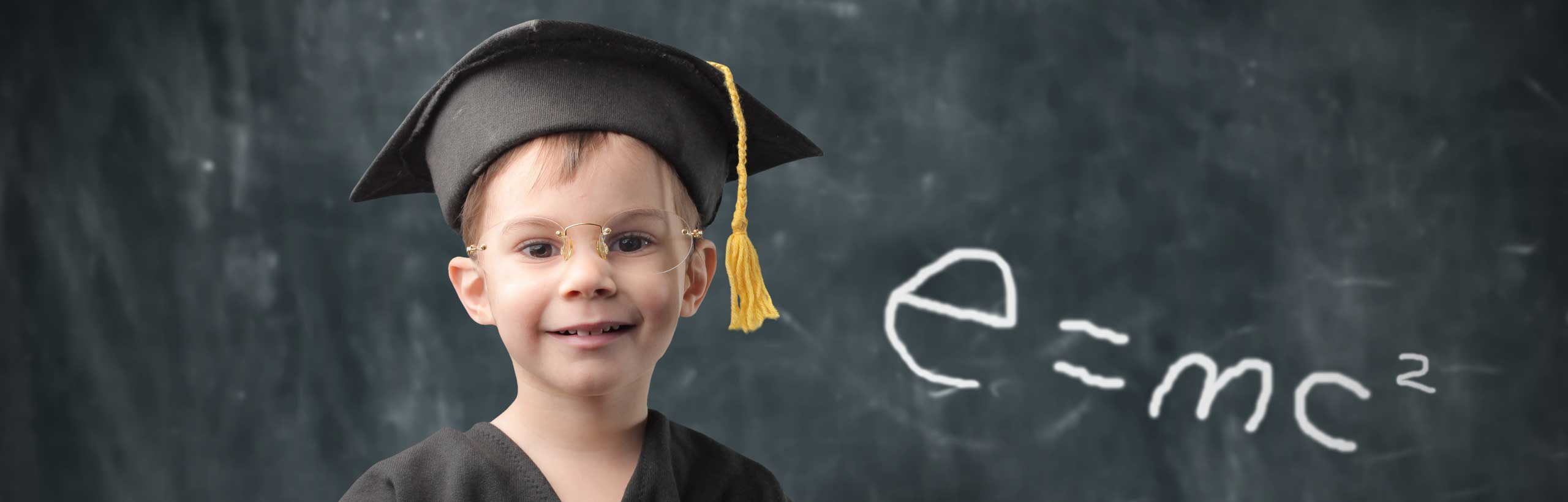# Solutions and Explanations to Intermediate Algebra Questions in Sample 1

Detailed solutions and full explanations of intermediate algebra questions in sample 6 are presented.

 (True or False)   ((22)3) = 25. Solution Evaluate ((22)3) and 25 . ((22)3) = 26 = 64 25 = 32 The statement "((22)3) = 25." is FALSE. (True or False)   The slope of a vertical line is undefined. Solution Let (2 , 5) and (2 , 7) being two points on the same vertical line since their x coordinates are both equal to 2. Let us find the slope through the two points. m = (7 - 5) / (2 - 2) = 2 / 0 = undefined. The statement "the slope of a vertical line is undefined" is TRUE. (True or False)   Two lines with positive slopes can be perpendicular. Solution For two lines to be perpendicular, the product of their slopes must be equal to - 1. If the two slopes are positive their product cannot be negative. Hence the statement "Two lines with positive slopes can be perpendicular" is FALSE. (True or False)   5 > 10 or 5 < 7. Solution The statement "5 > 10" is false and the statement "5 < 7" is true. The whole statement "5 > 10 or 5 < 7" is TRUE since there is an "or" and one of the two statements is true. (True or False)   The set of ordered pairs {(6,4),(2,-5),(-2,4),(6,-4)} is a function. Solution For the first coordinate 6 there are two possible second coordinates: 4 and - 4 and therefore the statement "the set of ordered pairs {(6,4),(2,-5),(-2,4),(6,-4)} is a function" is FALSE. (True or False)   The additive inverse of -10 is 10. Solution The additive inverse of a number x is. - x The additive inverse of - 10 is. - (-10) = 10 The statement "the additive inverse of -10 is 10" is TRUE. (True or False)   The product of two positive numbers is NOT positive. Solution The product of two positive numbers is positive and The statement "the product of two positive numbers is NOT positive" is FALSE. (True or False)   x + 2 = 7 is called an inequality. Solution x + 2 = 7 has an equal sign and is called an equation. Hence the statement "x + 2 = 7 is called an inequality" is FALSE. (True or False)   The associative property is used to write 4x + 8y = 4(x + 2y). Solution No. The distributive property is used to write 4x + 8y = 4(x + 2y) The statement "the associative property is used to write 4x + 8y = 4(x + 2y)" is FALSE (True or False)   The absolute value of a real negative number is negative. Solution The absolute value of a real negative number is positive (True or False)   -23 = (-2)3. Solution Evaluate each expression -23 = - 2 * 2 * 2 = - 8 (-2)3 = (-2)(-2)(-2) = - 8 (True or False)   30% of x is equal to 0.03x Solution 30% of x is written as 30% � x = (30/100) x = 0.03 x (True or False)   "x is at most equal to 9" is written mathematically as x < 9. Solution No. It is written as x ≤ 9 (True or False)   320 + 320 + 320 = 321 Solution factor and simplify 320 + 320 + 320 = 3�320 = 321 (True or False)   1.5 � 10-5 is the scientific notation of the number 0.0000015. Solution Convert 1.5 � 10-5 into decimal 1.5 � 10-5 = 1.5 / 105 = 1.5 / 100000 = 0.000015 (True or False)   1000/0 = 0. Solution Undefined; division by zero not allowed in mathematics. (True or False)   0/1000 = 0. Solution Zero divided by any non zero number gives zero (True or False)   0.00000010 = 1. Solution Any non zero number to the power zero gives 1. (True or False)   1 / (( -2 ) -4) = 16. Solution Rewrite 1 / (( -2 ) -4) with positive power. 1 / (( -2 ) -4) = (-2) 4 = (-2)(-2)(-2)(-2) = 16 (True or False)   x = 7 DOES NOT satisfy the inequality x - 7 < 0 Solution Substitute x by 7 in the inequality. 7 - 7 < 0 which gives 0 < 0 The above statement is false and therefore x = 7 does not satisfy the inequality.